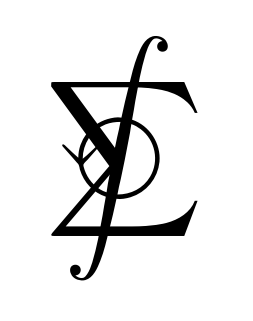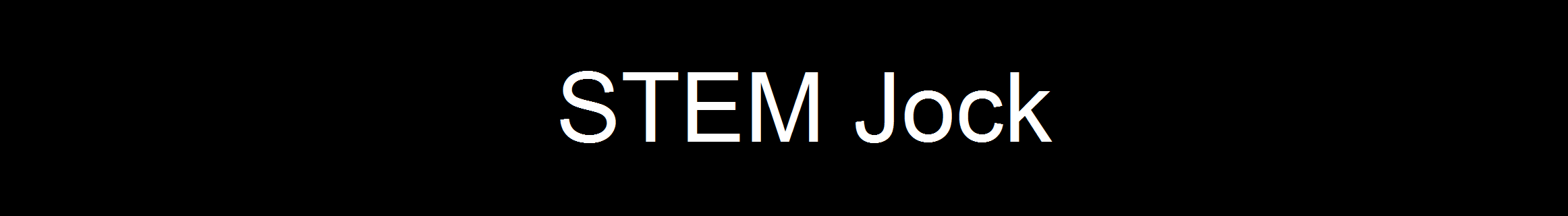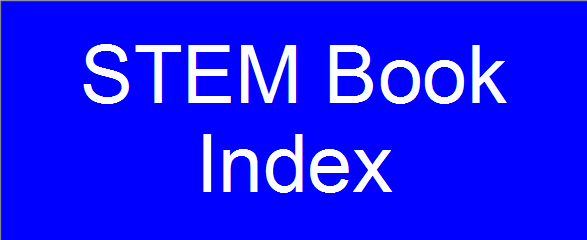On this webpage you will find my solutions to Chapters 6-11 of the eighth edition of "Calculus: Early Transcendentals" by James Stewart. Solutions to Chapters 1-5 are here, and solutions to Chapters 12-17 are here. Here is a link to the book's page on amazon.com. If you find my work useful, please consider making a donation. Thank you.

• Chapter 1: Functions and Models
• Section 1.1: Four Ways to Represent a Function
• Section 1.2: Mathematical Models: A Catalog of Essential Functions
• Section 1.3: New Functions from Old Functions
• Section 1.4: Exponential Functions
• Section 1.5: Inverse Functions and Logarithms

• Chapter 2: Limits and Differentiation
• Section 2.1: The Tangent and Velocity Problems
• Section 2.2: The Limit of a Function
• Section 2.3: Calculating Limits Using the Limit Laws
• Section 2.4: The Precise Definition of a Limit
• Section 2.5: Continuity
• Section 2.6: Limits at Infinity; Horizontal Asymptotes
• Section 2.7: Derivatives and Rates of Change
• Section 2.8: The Derivative as a Function

• Chapter 3: Differentiation Rules
• Section 3.1: Derivatives of Polynomials and Exponential Functions
• Section 3.2: The Product and Quotient Rules
• Section 3.3: Derivatives of Trigonometric Functions
• Section 3.4: The Chain Rule
• Section 3.5: Implicit Differentiation
• Section 3.6: Derivatives of Logarithmic Functions
• Section 3.7: Rates of Change in the Natural and Social Sciences
• Section 3.8: Exponential Growth and Decay
• Section 3.9: Related Rates
• Section 3.10: Linear Approximations and Differentials
• Section 3.11: Hyperbolic Functions

• Chapter 4: Applications of Differentiation
• Section 4.1: Maximum and Minimum Values
• Section 4.2: The Mean Value Theorem
• Section 4.3: How Derivatives Affect the Shape of a Graph
• Section 4.4: Indeterminate Forms and l'Hospital's Rule
• Section 4.5: Summary of Curve Sketching
• Section 4.6: Graphing with Calculus and Calculators
• Section 4.7: Optimization Problems
• Section 4.8: Newton's Method
• Section 4.9: Antiderivatives

• Chapter 5: Integrals
• Section 5.1: Areas and Distances
• Section 5.2: The Definite Integral
• Section 5.3: The Fundamental Theorem of Calculus
• Section 5.4: Indefinite Integrals and the Net Change Theorem
• Section 5.5: The Substitution Rule
• Chapter 6: Applications of Integration
• Section 6.1: Areas Between Curves
• Section 6.2: Volumes
• Section 6.3: Volumes by Cylindrical Shells
• Section 6.4: Work
• Section 6.5: Average Value of a Function

• Chapter 7: Techniques of Integration
• Section 7.1: Integration by Parts
• Section 7.2: Trigonometric Integrals
• Section 7.3: Trigonometric Substitution
• Section 7.4: Integration of Rational Functions by Partial Fractions
• Section 7.5: Strategy for Integration
• Section 7.6: Integration Using Tables and Computer Algebra Systems
• Section 7.7: Approximate Integration
• Section 7.8: Improper Integrals

• Chapter 8: Further Applications of Integration
• Section 8.1: Arc Length
• Section 8.2: Area of a Surface of Revolution
• Section 8.3: Applications to Physics and Engineering
• Section 8.4: Applications to Economics and Biology
• Section 8.5: Probability

• Chapter 9: Differential Equations
• Section 9.1: Modeling with Differential Equations
• Section 9.2: Direction Fields and Euler's Method
• Section 9.3: Separable Equations
• Section 9.4: Models for Population Growth
• Section 9.5: Linear Equations
• Section 9.6: Predator-Prey Systems

• Chapter 10: Parametric Equations and Polar Coordinates
• Section 10.1: Curves Defined by Parametric Equations
• Section 10.2: Calculus with Parametric Curves
• Section 10.3: Polar Coordinates
• Section 10.4: Areas and Lengths in Polar Coordinates
• Section 10.5: Conic Sections
• Section 10.6: Conic Sections in Polar Coordinates

• Chapter 11: Infinite Series
• Section 11.1: Sequences
• Section 11.2: Series
• Section 11.3: The Integral Test and Estimates of Sums
• Section 11.4: The Comparison Tests
• Section 11.5: Alternating Series
• Section 11.6: Absolute Convergence and the Ratio and Root Tests
• Section 11.7: Strategy for Testing Series
• Section 11.8: Power Series
• Section 11.9: Representations of Functions as Power Series
• Section 11.10: Taylor and Maclaurin Series
• Section 11.11: Applications of Taylor Polynomials
• Chapter 12: Vectors and the Geometry of Space
• Section 12.1: Three-Dimensional Coordinate Systems
• Section 12.2: Vectors
• Section 12.3: The Dot Product
• Section 12.4: The Cross Product
• Section 12.5: Equations of Lines and Planes
• Section 12.6: Cylinders and Quadric Surfaces

• Chapter 13: Vector Functions
• Section 13.1: Vector Functions and Space Curves
• Section 13.2: Derivatives and Integrals of Vector Functions
• Section 13.3: Arc Length and Curvature
• Section 13.4: Motion in Space: Velocity and Acceleration

• Chapter 14: Partial Derivatives
• Section 14.1: Functions of Several Variables
• Section 14.2: Limits and Continuity
• Section 14.3: Partial Derivatives
• Section 14.4: Tangent Planes and Linear Approximations
• Section 14.5: The Chain Rule
• Section 14.6: Directional Derivatives and the Gradient Vector
• Section 14.7: Maximum and Minimum Values
• Section 14.8: Lagrange Multipliers

• Chapter 15: Multiple Integrals
• Section 15.1: Double Integrals over Rectangles
• Section 15.2: Double Integrals over General Regions
• Section 15.3: Double Integrals in Polar Coordinates
• Section 15.4: Applications of Double Integrals
• Section 15.5: Surface Area
• Section 15.6: Triple Integrals
• Section 15.7: Triple Integrals in Cylindrical Coordinates
• Section 15.8: Triple Integrals in Spherical Coordinates
• Section 15.9: Change of Variables in Multiple Integrals

• Chapter 16: Vector Calculus
• Section 16.1: Vector Fields
• Section 16.2: Line Integrals
• Section 16.3: The Fundamental Theorem for Line Integrals
• Section 16.4: Green's Theorem
• Section 16.5: Curl and Divergence
• Section 16.6: Parametric Surfaces and Their Areas
• Section 16.7: Surface Integrals
• Section 16.8: Stokes' Theorem
• Section 16.9: The Divergence Theorem

• Chapter 17: Second-Order Differential Equations
• Section 17.1: Second-Order Linear Equations
• Section 17.2: Nonhomogeneous Linear Equations
• Section 17.3: Applications of Second-Order Differential Equations
• Section 17.4: Series Solutions
Section 6.1 Section 6.2 Section 6.3
Exercise 1 Exercise 1 Exercise 1 Exercise 26 Exercise 51
Exercise 2 Exercise 2 Exercise 2 Exercise 27 Exercise 52
Exercise 3 Exercise 3 Exercise 3 Exercise 28 Exercise 53
Exercise 4 Exercise 4 Exercise 4 Exercise 29 Exercise 54
Exercise 5 Exercise 5 Exercise 5 Exercise 30 Exercise 55
Exercise 6 Exercise 6 Exercise 6 Exercise 31 Exercise 56
Exercise 7 Exercise 7 Exercise 7 Exercise 32 Exercise 57
Exercise 8 Exercise 8 Exercise 8 Exercise 33
Exercise 9 Exercise 9 Exercise 9 Exercise 34
Exercise 10 Exercise 10 Exercise 10 Exercise 35
Exercise 11 Exercise 11 Exercise 11 Exercise 36
Exercise 12 Exercise 12 Exercise 12 Exercise 37
Exercise 13 Exercise 13 Exercise 13 Exercise 38
Exercise 14 Exercise 14 Exercise 14 Exercise 39
Exercise 15 Exercise 15 Exercise 15 Exercise 40
Exercise 16 Exercise 16 Exercise 16 Exercise 41
Exercise 17 Exercise 17 Exercise 17 Exercise 42
Exercise 18 Exercise 18 Exercise 18 Exercise 43
Exercise 19 Exercise 19 Exercise 19 Exercise 44
Exercise 20 Exercise 20 Exercise 20 Exercise 45
Exercise 21 Exercise 21 Exercise 21 Exercise 46
Exercise 22 Exercise 22 Exercise 22 Exercise 47
Exercise 23 Exercise 23 Exercise 48
Exercise 24 Exercise 24 Exercise 49
Exercise 25 Exercise 50

## Chapter 7 - Techniques of Integration

### Problems Plus

Statement | Solution - Problem 2
Statement | Solution - Problem 11
Statement | Solution - Problem 14
Statement | Solution - Problem 16

## Chapter 9 - Differential Equations

### Section 9.3 - Separable Equations

Statement | Solution - Exercise 29
Statement | Solution - Exercise 30
Statement | Solution - Exercise 31
Statement | Solution - Exercise 32
Statement | Solution - Exercise 33
Statement | Solution - Exercise 34
Statement | Solution - Exercise 35
Statement | Solution - Exercise 36
Statement | Solution - Exercise 37
Statement | Solution - Exercise 38
Statement | Solution - Exercise 39
Statement | Solution - Exercise 40
Statement | Solution - Exercise 41
Statement | Solution - Exercise 42
Statement | Solution - Exercise 43
Statement | Solution - Exercise 44

### Problems Plus

Statement | Solution - Problem 1
Statement | Solution - Problem 2
Statement | Solution - Problem 3
Statement | Solution - Problem 4
Statement | Solution - Problem 5
Statement | Solution - Problem 6
Statement | Solution - Problem 7
Statement | Solution - Problem 8
Statement | Solution - Problem 9
Statement | Solution - Problem 10
Statement | Solution - Problem 11

## Chapter 11 - Infinite Sequences and Series

### Problems Plus

Statement | Solution - Problem 1
Statement | Solution - Problem 2
Statement | Solution - Problem 3
Statement | Solution - Problem 4
Statement | Solution - Problem 6
Statement | Solution - Problem 7
Statement | Solution - Problem 8
Statement | Solution - Problem 9
Statement | Solution - Problem 10
Statement | Solution - Problem 13
Statement | Solution - Problem 14
Statement | Solution - Problem 17
Statement | Solution - Problem 19
Statement | Solution - Problem 21
Statement | Solution - Problem 22
Statement | Solution - Problem 25